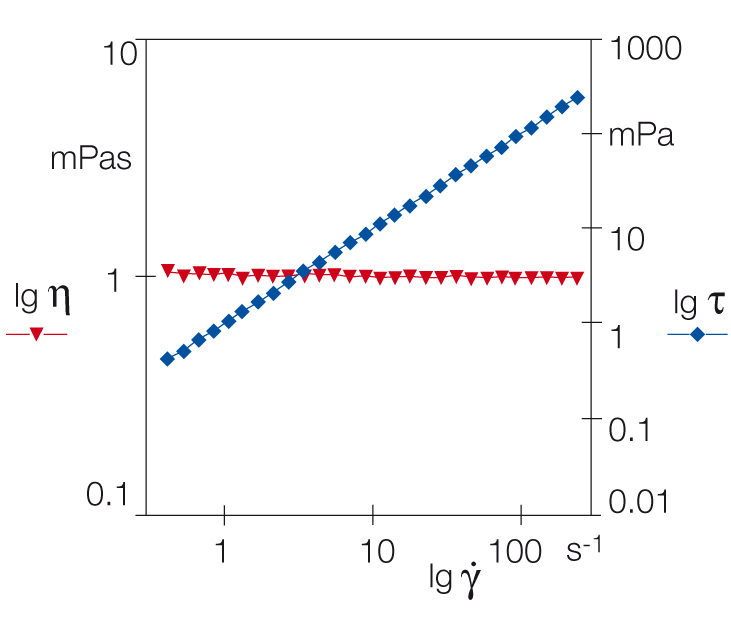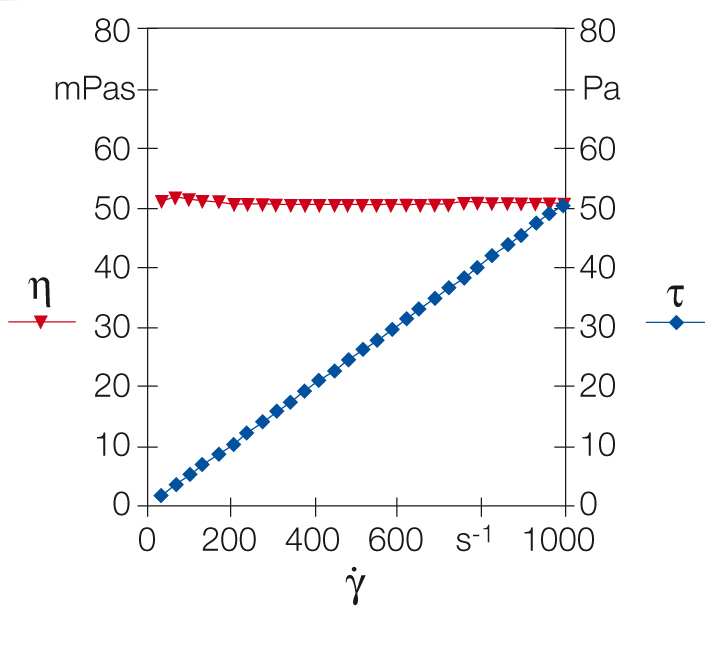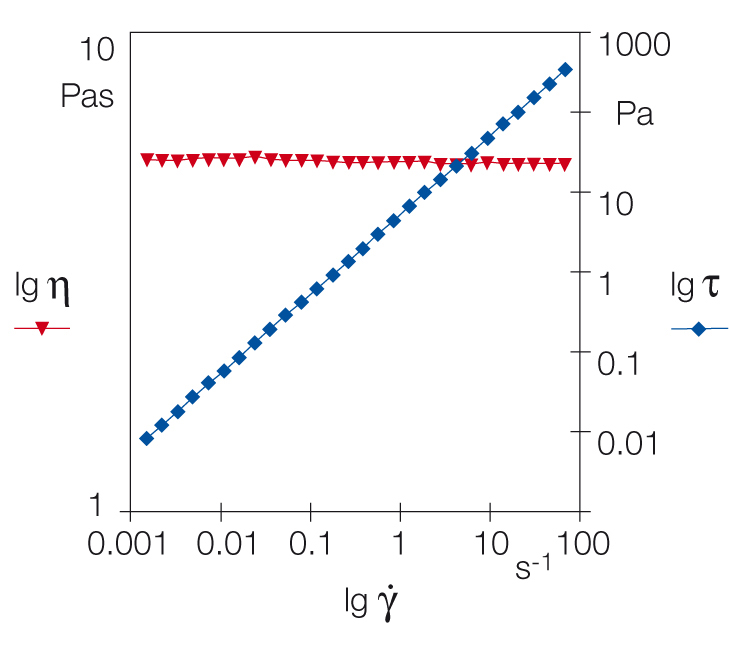19 Rates

# Examples of a flow curve and viscosity curve

Typical examples for ideally viscous, Newtonian liquids are water, mineral oil, silicone oil, salad oil, solvents such as acetone, as well as viscosity standards (e.g. calibration oils).

Water shows a constant viscosity of η = 1 mPas, which is ideally viscous behavior (Figure 1). The presentation in the diagram was limited to a shear-rate range of between 0.2 s-1 and 200 s-1. Mineral oil and silicone oil show similar behavior (Figures 2 and 3).

At lower shear rates, the detectable torque signal would be too small under these measuring conditions. At higher shear rates, turbulent flow effects in the gap would cause disturbances to the flow. There is no way of preventing such effects when testing fast-flowing low-viscosity liquids. In this case, the requirements for a scientifically precise calculation of the viscosity would not be met because the precondition is laminar flow.Figure 1: Flow curve and viscosity curve of water, tested in a double-gap system at T = +20 °C: Within the shear rate range shown (0.2 s-1 to 200 s-1), water displays ideally viscous flow behavior with η = 1 mPas.Figure 2: Flow curve and viscosity curve of a mineral oil, presented on a linear scale. Across the entire measuring range, the viscosity of the mineral oil remains constant, thus showing ideally viscous flow behavior.Figure 3: Flow curve and viscosity curve of an ideally viscous silicone oil, presented on a logarithmic scale for better illustration of the values in the low shear range.